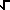Home    |    Teacher    |    Parents    |    Glossary    |    About UsEmail this page to a friendResources· Cool Tools · Formulas & Tables · References · Test Preparation · Study Tips · Wonders of MathSearchComplexity (Math | Miscellaneous | Complexity | 1/i)

### Rationale that 1/i = -i

We know that by definition
i =( -1 )
Similarly,
i*i =( -1 ) *( -1 ) =( -1 )2 = -1

By algebra we get:

i*i = -1
-i*i = 1     (multiply both sides by -1)
-i = 1/i     (divide both sides by i)